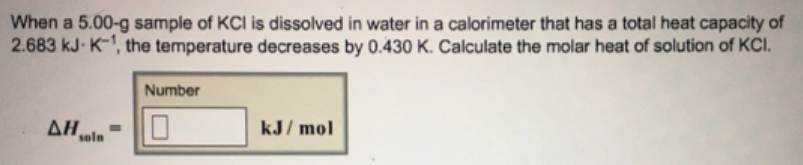# Problem: When a 5.00-g sample of KCI is dissolved in water in a calorimeter that has a total heat capacity of 2.683 kJ • K-1, the temperature decreases by 0.430 K. Calculate the molar heat of solution of KCI.

###### FREE Expert Solution
89% (425 ratings)###### Problem Details

When a 5.00-g sample of KCI is dissolved in water in a calorimeter that has a total heat capacity of 2.683 kJ • K-1, the temperature decreases by 0.430 K. Calculate the molar heat of solution of KCI.